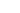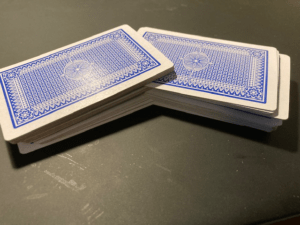### Tag: riffle## How to calculate how effective a Riffle is

In my previous post I mentioned about writing a program to determine how effective a riffle shuffle was. So here’s the code.

### How it works

I’m using an array of 52 chars to hold the deck. I’m only interested in the card’s position in the deck so each card is initialised with a value in the range 0-51. I’m using three other similar sized arrays.

• tempCards are used purely for doing the riffle.
• distances are used to calculate the maximum distance the card moved
• startPos tracks the cards starting position 0-51 before doing lots of rounds of riffles.

The program starts by picking up ( as a parameter) how many rounds you want it to run. It defaults to 10 if no value is input.

It then clears distances and inits cards. In each round, it starts by storing card positions in startPos. It then does seven riffles and works out how far a card has moved. If it has moved more than before (in distances) it stores it in distances.

DoRiffle works by indexing through the two 1/2 deck piles taking a card from each and a 50:50 random chance determines which of the two cards goes into the shuffled deck first and then second.

Here’s the listing.

``````// riffle.c by D. Bolton (C) 2020 Learncgames.com - TYou are free to redistribute but please keep this line in

#include <math.h>
#include <stdio.h>
#include <stdlib.h>
#include <string.h>
#include <time.h>

// #defines
#define MAXROUNDS 10
#define NUMRIFFLES 7
#define NUMCARDS 52

// variables
int NumRounds;
char cards[NUMCARDS],tempCards[NUMCARDS],startPos[NUMCARDS];
int distances[NUMCARDS];
time_t t;

// functions

// Convert string to int calling strtol
int GetIntArg(char* str) {
char* ptr;
return strtol(str, &ptr, 10);
}

// Merges two cards a and b. 50:50 chance that a above b or other way
void DoRiffle() {
// Copy cards into tempCards
memcpy(tempCards, cards, sizeof(cards));
// Merge each pair of cards tempCards[i] and TempCards[i+26]
int cardIndex = 0;
for (int i = 0; i < NUMCARDS / 2; i++) {
if (rand() % 2) {
cards[cardIndex++] = tempCards[i];
cards[cardIndex++] = tempCards[i + 26];

}
else {
cards[cardIndex++] = tempCards[i + 26];
cards[cardIndex++] = tempCards[i];
}
}
}

// Works out how far cards have moved, added to distances
void CalculateDistances() {
for (int i = 0; i < NUMCARDS; i++) {
int moved = abs(cards[i] - startPos[i]);
if (moved > distances[i])
distances[i] = moved;
}
}

void DoShuffles() {
// Clear distances and init cards
for (char i = 0; i < NUMCARDS; i++) {
distances[i] = 0;
cards[i] = i;
}
// do Numrounds  rounds
for (int round =0;round<NumRounds;round++){
// Mark where the card starts
for (char i = 0; i < NUMCARDS; i++) {
startPos[i] = cards[i];
}
// Do seven riffles
for (int i = 0; i < NUMRIFFLES; i++) {
DoRiffle();
}
CalculateDistances();
}
int furthest = 0;
for (int i = 0; i < NUMCARDS; i++) {
printf("Distance[%d]=%d\n", i, distances[i]);
if (distances[i]> furthest) {
furthest = distances[i];
}
}
printf("furthest moved is %d\n", furthest);
}

int main(int argc, char* argv[]) {
srand((unsigned)time(&t));
if (argc ==1 || argc== 2) {
NumRounds = MAXROUNDS;
if (argc == 2) {
NumRounds = GetIntArg(argv);
printf("Numrounds = %d\n", NumRounds);
}
DoShuffles();
}
else {
printf("Please supply 0 or 1 arguments e.g. riffle 60\n");
}
}``````

Even with ten rounds I’ve seen cards move 51 positions. With 5,000 rounds all cards but one moved by 51 and one by 50.## How many riffles are needed to shuffle a deck?It fascinates me because 52! is such a large number.  Here it is in full 8.0658* 10^67 or 80,658,175,170,943,878,571,660, 636,856,403,766,975,289,505,440,883, 277,824,000,000,000,000. That is the possible number of ways of shuffling a pack of cards.

It means that when you shuffle a deck of cards, it’s possible that you are the first person on Earth to ever get that particular arrangement. It’s the kind of fact that amazes me. Another one is that it takes a very long time for particles emitted from the centre of the sun to reach the surface and blast into space. On the order of many many years. (Thousands of years!)

Playing cards have only been around maybe 500 years as we know it (52 card deck) though date back to 9th century China for their invention. If there had been a billion shuffles each day during that 500 years, that’s only 1.8 x 10^14 shuffles. That is a minuscule fraction of the possible number of arrangements so the chances are that any shuffled arrangement is new is pretty high.

It’s accepted that seven is the number of riffles needed to perfectly shuffle a pack of cards. A riffle is where you split the deck in two and then merge the two halves back into one deck as in the photo I took.

I proved this once by writing a program to simulate riffles  and looking how far cards have moved after seven. In fact a card at the top of the deck moved to the bottom after only six riffles. I’ll try and write that in C and will publish it here in a day or two.

Other shuffling techniques like smooshing (spreading out all the cards on the table with their backs face up and then pushing them together) are nowhere near as efficient. It’s estimated it can take thousands of smooshes to properly shuffle a pack. It’s not easy to simulate, though one of these days I’ll have a go and see if I can come up with a more accurate estimation.